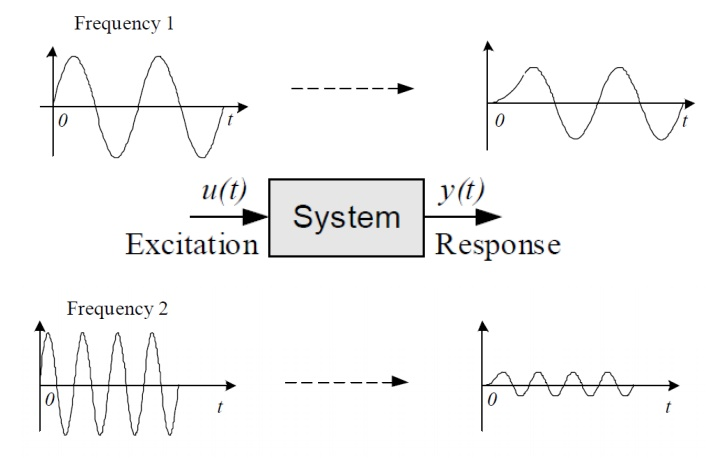Home | | Control System Engineering | Frequency Response

# Frequency ResponseThe frequency response of a system is a frequency dependent function which expresses how a sinusoidal signal of a given frequency on the system input is transferred through the system.

Frequency Response

The frequency response of a system is a frequency dependent function which expresses how a sinusoidal signal of a given frequency on the system input is transferred through the system. Time-varying signals at least periodical signals —which excite systems, as the reference (set point) signal or a disturbance in a control system or measurement signals which are inputs signals to signal filters, can be regarded as consisting of a sum of frequency components. Each frequency component is a sinusoidal signal having certain amplitude and a certain frequency. (The Fourier series expansion or the Fourier transform can be used to express these frequency components quantitatively.) The frequency response expresses how each of these frequency components is transferred through the system. Some components may be amplified, others may be attenuated, and there will be some phase lag through the system.

The frequency response is an important tool for analysis and design of signal filters (as low pass filters and high pass filters), and for analysis, and to some extent, design, of control systems. Both signal filtering and control systems applications are described (briefly) later in this chapter. The definition of the frequency response — which will be given in the next section — applies only to linear models, but this linear model may very well be the local linear model about some operating point of a non-linear model. The frequency response can found experimentally or from a transfer function model. It can be presented graphically or as a mathematical function.Study Material, Lecturing Notes, Assignment, Reference, Wiki description explanation, brief detail
Control Systems : Frequency Response Analysis : Frequency Response |